## A conductor carrying a current I = 16.5 A is directed along the positive x axis and perpendicular to a uniform magnetic field. A magnetic fo

Question

A conductor carrying a current I = 16.5 A is directed along the positive x axis and perpendicular to a uniform magnetic field. A magnetic force per unit length of 0.130 N/m acts on the conductor in the negative y direction. (a) Determine the magnitude of the magnetic field in the region through which the current passes.

in progress 0
2 months 2021-07-22T20:24:07+00:00 1 Answers 9 views 0

1. To solve this problem we will apply the concepts related to the Magnetic Force, this is given by the product between the current, the body length, the magnetic field and the angle between the force and the magnetic field, mathematically that is,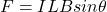Here,

I = Current

L = Length

B = Magnetic Field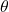= Angle between Force and Magnetic Field

But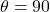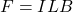Rearranging to find the Magnetic Field,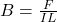Here the force per unit length,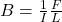Replacing with our values,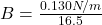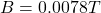Therefore the magnitude of the magnetic field in the region through which the current passes is 0.0078T